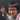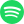# Perfectly Competitive Markets in Math

June 26, 2020 · 5:53 am · EconMath

Perfect Competition, or as I like to abbreviate it, Perfekt, is your standard paired Supply vs Demand market graph along with a firm graph where Marginal Revenue = Demand = Average Revenue = Price.

You can interact with it below: (See this page to interact with only this applet)

Mode

$p_{mkt} =60$

$p_{eq} =60.85119791515859$

$p_{min} =55.14677965740265$

Profit per unit: $p_{eq} - p_{mkt} = 0.8511979151585933$

Economic state? Approximately Normal Profit

Should the firm shutdown in the short run? No.

## Definitions

$C_S = S(0)$ is the intersection between $S$ and the y-axis.

$C_D = D(0)$ is the intersection between $D$ and the y-axis.

$C_{MC}$ is an arbitrary constant that shifts $MC$ up or down.

$p_{mkt}$ is the market price.

$p_{eq}$ is the profit-maximizing price.

$p_{min}$ is the minimum of $AVC$.

## The default $MC$ function is awfully complicated!!

Yup, I was having trouble finding a decent function such that all of the cool things like the intersection between $P$ and $ATC$ and $P_{mkt}$ and $AVC$, so I asked Taiga to engineer one using Desmos:

The final function is very cursed:

$MC(q) = \frac{4.4(0.134q + 4.7)^2 - 12(0.134q + 4.7) + 50}{3.2(0.134q + 4.7) - 13.14} 8.4 - 125$

### Thought Process

The equation was written so that it could be manipulated as easily as possible. We already knew the general shape was going to have a sharp descent from the positive y-axis, and then slowly “climb up”. The “climb up” part could be approximated by a function that has a slant asymptote. Therefore, we began with a rational function with a quotient whose degree was 1 for a nice linear asymptote. This is why the numerator looks like a quadratic function (think of $0.134q + 4.7$ as $X$) and the denominator looks linear. Then, to perform linear transformations, we replaced $X$ with $mq + b$ for horizontal stretching/squishing and shifting and took the entire function and replaced it with $MR + B$ for vertical stretching/squishing and shifting.

## Why?

As I was watching Matt Pedlow’s AP Microeconomics series for this year’s exam, I noticed that we can actually define many of the relationships between quantity and price using discrete calculus. So I thought it would be interesting to talk about how that would span out.

Also, it’s always nice to be able to have an interactive visualization of things, and I haven’t seen anything for microeconomics anywhere else.

## Mathematical Formalization

Let me show you how some of these functions are calculated from $MC$.

### Defining $TC$ in terms of $MC$

The definition of Marginal Cost ($MC$) is the difference in the Total Cost ($TC$) of producing the currenth $n$th good minus the $TC$ of producing the last good, $(n-1)$th. Using notation from my post on discrete derivatives, we can define $MC$ as so:

\begin{aligned} MC(q) &\coloneqq TC(q) - TC(q - 1) \\ &= \Delta TC(q-1) \\ MC(q + 1) &= \Delta TC(q) \\ MC(q+1) \Delta I(q) &= \Delta TC(q) \\ \sum_{q = 0}^q MC(q + 1) \Delta I(q) &= \sum_{q = 0}^q \Delta TC(q) \\ &= TC(q) - TC(0) \\ TC(q) &= TC(0) + \sum_{q = 0}^q MC(q + 1) \Delta I(q) \\ \end{aligned}

By the way, both $MC$ and $TC$ map $Q \to P$, where $Q$ is the set of nonnegative integers,1 and $P$ is the set of reals with a unit of economic value, such as dollars.2 (In the app above, $Q$ has a different meaning: the domain that will be plotted in the graph.)

As a side note, we define a special case for $MC(0) \coloneqq TC(0)$ since the general definition results in a term $TC(-1)$, even though $-1 \notin Q$.

### Defining $ATC$ in terms of $MC$

We can define Average Total Cost ($ATC$) similarly, but beware that since it is calculated with respect to the quantity of goods, its domain is actually $Q - \{0\}$. That is, it doesn’t make sense to talk about the average cost per good when no goods have been produced. So, $ATC \colon Q-\{0\} \to P$, and

$ATC(q) \coloneqq \frac{TC(q)}{q}$

We can also define $MC$ in terms of $ATC$:

\begin{aligned} ATC(q) &= \frac{TC(q)}{q} \\ q \cdot ATC(q) &= TC(q) \\ \Delta [q \cdot ATC](q) &= \Delta TC(q) \\ q \Delta ATC(q) &= MC(q + 1) \\ MC(q) &= (q + 1) \Delta ATC(q + 1) \\ \end{aligned}

## Market Price

Since algebraically finding the market price is hard, this approximates by finding the closest quantity $q_{mkt}$ such that

$p_{mkt} \coloneqq S(q_{mkt}) = D(q_{mkt}).$

And if

$p_{eq} \coloneqq MC(q_{eq}) = ATC(q_{eq})$

is close enough to $p_{mkt}$, about $1$ unit, then we’ll say the firm is making approximately normal profit.

Normally, the firm tries to produce at the price $p_c = MR(q_c) = MC(q_c)$. For Perfekt firms, $MR(q) = p_{mkt}$; that is, they are price takers. So,

$p_c = p_{mkt} = MC(q_c).$

We can compare $p_{eq}$ and $p_c$ to find the sign of the economic profit the firm is making:

ComparisonEconomic State
$p_c < p_{eq}$Economic Loss
$p_c = p_{eq}$Normal Profit
$p_c > p_{eq}$Economic Profit

## Shutdown Point

If the firm is making an economic loss, we can also find

$p_{min} \coloneqq \min_{q \in Q} (AVC(q))$

and compare $p_{c}$ and $p_{min}$ like so:

ComparisonShould the firm shutdown?
$p_{min} < p_{c}$No
$p_{min} = p_{c}$Doesn’t Matter3
$p_{min} > p_{c}$Yes

1. The visualization connects points with consecutive quantities with a line segment, which is why the curves look continuous.
2. Note that this definition is not formal.
3. With respect to loss minimization.Streaming direct thought dumps from Yuto Nishida.
Connect with me on LinkedIn! I'm currently listening to: